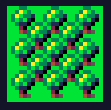## Rendering a tilemap in the tile renderer

In this part we continue with the code from rendering a single tile and implement rendering multiple tiles with the tile renderer through a so called tilemap. This can be used to draw the background of your game for example. The tileset that is being rendered is from Aleksandr Makarov at itch.io. See adding media resources as an example on how to add the tileset png to the project.

The TileMap is implemented as a 2D array of integers. The first index represents the row and the second index the column. The integer value represents the tile ID, i.e. which tile to render from the TileSet. The integer could be replaced by a Tile class. The Tile class could then keep track of the state of the tile and keep track of actions to perform.

```    public class TileMap
{
public TileMap(int numRows, int numColumns)
{
NumRows = numRows;
NumColumns = numColumns;

Tiles = new int[NumRows][];

for (var i = 0; i < NumRows; ++i)
Tiles[i] = new int[NumColumns];
}

public int this[int row, int col]
{
get { return Tiles[row][col]; }
set { Tiles[row][col] = value; }
}

private int[][] Tiles { get; }

public int NumRows { get; }

public int NumColumns { get; }
}
```

The TileRenderer is the class responsible for rendering the TileMap with the associated TileSet. It contains a reference to the TileSet from which to render the tiles as specified in the TileMap. The RenderMap method is responsible for drawing the tile map to the canvas. When looping over the rows and columns of the tile map it is best to first loop over the rows and in the inner loop go over the columns. The physical memory of the device is linear and when looping over the columns the access follows the physical memory layout which gives a better performance.

```    public class TileRenderer
{
private SKRect sourceRect;
private SKRect targetRect;

public int TileScaling { get; set; } = 4;

public TileSet ActiveTileSet { get; set; }

public int RenderTileSize => TileScaling * ActiveTileSet.TileSize;

public void RenderMap(float x, float y, TileMap map, SKCanvas canvas)
{
var x0 = x;

for (int i=0;i < map.NumRows;++i)
{
x = x0;

for(int j=0;j < map.NumColumns;++j)
{
var tileId = map[i, j];

// Split tileId into row and column of the tile set
var row = tileId / ActiveTileSet.NumTilesX;
var col = tileId - row * ActiveTileSet.NumTilesX;

RenderTile(x, y, col, row, canvas);

x += RenderTileSize;
}

y += RenderTileSize;
}
}

public void RenderTile(float x, float y, int tx, int ty, SKCanvas canvas)
{
var sourceTileSize = ActiveTileSet.TileSize;
sourceRect.Left = tx * sourceTileSize;
sourceRect.Right = sourceRect.Left + sourceTileSize;
sourceRect.Top = ty * sourceTileSize;
sourceRect.Bottom = sourceRect.Top + sourceTileSize;

var targetTileSize = TileScaling * sourceTileSize;
targetRect.Left = x;
targetRect.Right = targetRect.Left + targetTileSize;
targetRect.Top = y;
targetRect.Bottom = targetRect.Top + targetTileSize;

canvas.DrawBitmap(ActiveTileSet.TilesBitmap, sourceRect, targetRect);
}
}
```

Finally, the TileMap, TileSet and the TileRenderer are constructed in the Init method of the PixelApp and the TileMap is drawn in the Render method with the TileRenderer. Note, that initializing the TileMap is quite a bit of code even though the map is just of size 3 * 3. Normally, the tile map is created by a level editor, which saves the TileMap to a file. The file is then loaded and parsed to create the TileMap. This results in less code and it is way easier to create a visually pleasing map from a level editor.

```    public class PixelApplication
{
private SKColor _fillColor = new SKColor(20, 20, 40);
private double width;
private double height;
TileSet tileSet;
TileRenderer tileRenderer;
TileMap tileMap;

public void Init(float w, float h)
{
width = w;
height = h;

// https://iknowkingrabbit.itch.io/mas-grass-land
string resourceID = "pixelapp.Media.tileset.png";

tileSet = new TileSet(resourceID, 8);
tileRenderer = new TileRenderer { ActiveTileSet = tileSet, TileScaling = 4 };
tileMap = new TileMap(3, 3);

// Column 0, Row 4 Single Tree
int treeTile = 4 * tileSet.NumTilesX + 0;
int forestTile00 = 4 * tileSet.NumTilesX + 1;
int forestTile01 = 4 * tileSet.NumTilesX + 2;
int forestTile02 = 4 * tileSet.NumTilesX + 3;

int forestTile10 = 5 * tileSet.NumTilesX + 1;
int forestTile11 = 5 * tileSet.NumTilesX + 2;
int forestTile12 = 5 * tileSet.NumTilesX + 3;

int forestTile20 = 6 * tileSet.NumTilesX + 1;
int forestTile21 = 6 * tileSet.NumTilesX + 2;
int forestTile22 = 6 * tileSet.NumTilesX + 3;

tileMap[0, 0] = forestTile00;
tileMap[0, 1] = forestTile01;
tileMap[0, 2] = forestTile02;

tileMap[1, 0] = forestTile10;
tileMap[1, 1] = forestTile11;
tileMap[1, 2] = forestTile12;

tileMap[2, 0] = forestTile20;
tileMap[2, 1] = forestTile21;
tileMap[2, 2] = forestTile22;
}

public void Render(SKCanvas canvas, double updateDelta)
{
canvas.Clear(_fillColor);

tileRenderer.RenderMap((float)width / 2.0f, (float)height / 2.0f, tileMap, canvas);
}
}
```

A tilemap showing a forest should be rendered just like the image below.